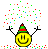# Acceleration due to gravity

• Gurasees
In summary, the problem is to determine which ball, thrown straight up and straight down from the top of a building with the same speed, has the greater speed when it reaches the ground. The student's attempt at a solution is that the ball thrown straight up will have a greater speed due to having a longer acceleration time. However, this answer is incorrect and the problem can be solved conceptually without using mathematical equations.

#### Gurasees

Problem Statement

From the top of the building if we throw one ball straight up with speed v and one ball straight down with the same speed v, then which ball has the greater speed when it reaches the ground?

The attempt at a solution

I think the ball which is first thrown straight up will have greater speed (than the ball which is thrown straight down) when they reach the ground because it has had accelerated for longer time than the second ball.

Last edited by a moderator:
Gurasees said:
Problem Statement

From the top of the building if we throw one ball straight up with speed v and one ball straight down with the same speed v, then which ball has the greater speed when it reaches the ground?

The attempt at a solution

I think the ball which is first thrown straight up will have greater speed (than the ball which is thrown straight down) when they reach the ground because it has had accelerated for longer time than the second ball.
You deleted the part of the Homework Template that asks for the "Relevant Equations" -- you should use the equation for the vertical motion of that mass to figure this problem out. The answer may not be intuitive to you yet, so it's best to do the math...

Probably you should not guess, but try to formulate the problem mathematically. Do you have any idea which formulas you could use to solve it? If you are not familiar with these kind of calculations yet I suggest to start easy. What's the velocity of the ball, when touching the ground if ##v_0=0##?

•Chestermiller
Without doing the actual calculations, can you describe how the first ball's velocity changes going up and compare that with how its velocity changes coming back down to you at the top of the building? Then you can compare that ball continuing down from the top of the building with the second ball you throw down from the same point.

In case you haven't figured it out yet from the other responses, your answer, OP, was wrong.

•FactChecker
stockzahn said:
Probably you should not guess, but try to formulate the problem mathematically. Do you have any idea which formulas you could use to solve it? If you are not familiar with these kind of calculations yet I suggest to start easy. What's the velocity of the ball, when touching the ground if ##v_0=0##?

Actually, as @FactChecker has implied, this can be solved conceptually without having to resort to solving the mathematics.

@Gurasees : For the ball that you tossed vertically upwards, what do you think its speed will be on the way down when it passes its original location?

Zz.

•FactChecker
ZapperZ said:
Actually, as @FactChecker has implied, this can be solved conceptually without having to resort to solving the mathematics.
But I LIKE the math!•FactChecker and phinds
berkeman said:
But I LIKE the math!Doesn’t mean you can’t solve it that way, but it can be solved without it.

This is the type of question that I give the students when we employ peer-instruction technique.

Zz.

•berkeman